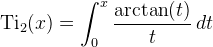Computes the inverse tangent integral.

Namespace:  Meta.Numerics.Functions
Assembly:  Meta.Numerics (in Meta.Numerics.dll) Version: 4.1.4Syntax
```public static double IntegralTi(
double x
)```

#### Parameters

x
Type: SystemDouble
The argument.

#### Return Value

Type: Double
The value of Ti2(x).Remarks

The inverse tangent integral is defined by:See Also